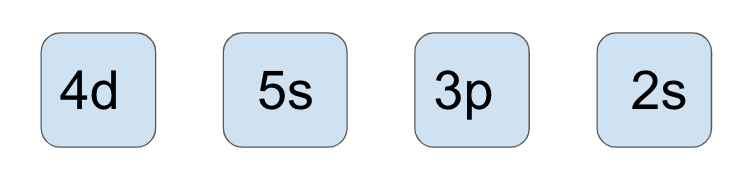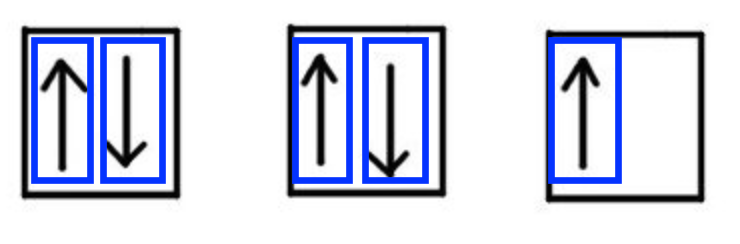# Problem: Identify the orbital represented by the quantum numbers given below:n = 3                    l = 1                                m      l = 0                        m   s = -1/2 Now select the electron represented by the quantum numbers.

###### Problem Details

Identify the orbital represented by the quantum numbers given below:

n = 3                    l = 1                                m      l = 0                        m   s = -1/2Now select the electron represented by the quantum numbers.